Revision 4 as of 2007-05-07 05:42:46

# Assignment 4: Investigating Importance Sampling

## Description

Rendering requires the computation of many different types of integrals. For example, in the last assignment we numerically integrated over the back element of the realistic camera lens to compute the irradiance incident on each pixel of your virtual camera's sensor. In this assignment you will take a close look at how pbrt computes the radiance reflected from a surface in a certain direction by integrating the surface's reflection function (BRDF) against incoming light from all directions.

pbrt numerically estimates the value of this integral via Monte Carlo integration. As you are now well aware, the variance of a Monte Carlo estimator manifests itself as noise in a rendered image. Thus, when integrating a function, it is important to choose samples from the domain of integration such that variance in the integral estimate is minimized. Importance sampling is a variance reduction technique that draws samples using a distribution that is proportional to the value of the function over the domain. In this assignment you will explore various approaches for sampling irradiance on scene objects due to an infinite area light source (environment light) and explain why and when certain approaches are preferable to others.

We are happy to inform you that this assignment is designed to be significantly shorter than Assignments 2 or 3.

Read chapters 13 (especially 13.5), 14 (especially 14.3.4) and 15 (especially 15.6) in the pbrt book. This assignment will require a solid understanding of importance sampling, so please read the these chapters carefully.

Notice that there is a typo in the MULTIPLE IMPORTANCE SAMPLING EQUATION on pbrt page 676. The pbrt code core/transport.cpp is correct, the equation in the book is wrong. Instead of multiplying both summations by the factor 1/(nf + ng) as given in the book, the first summation (sum over samples drawn from a pdf from f) should be scaled by 1/nf. The second summation should be scaled by 1/ng. A correction is posted on the pbrt Errata page.

## Step 2: Importance Sampling an Infinite Area Light

In the scenes we've rendered in this class so far, objects were lit using a small number of spotlight or area light sources. In the real world, light incident on an object comes not just from light emitters, but from all directions. One way of approximating this effect in pbrt is to use an environment light (infinite area light in pbrt), which is used to define light entering the scene from all directions due the surrounding environment. Environment lighting can greatly contribute to the realism and richness of your renderings, however, since computing environment lighting involves integrating incoming radiance over the entire hemisphere above a surface normal, in the worst case, Monte Carlo estimates are prone to be noisy, and require many samples to produce images of reasonable quality. In the scene below, images of the killeroo scene are rendered with 4, 8, and 128 samples per pixel.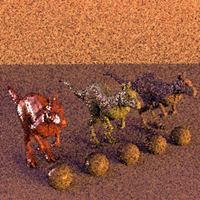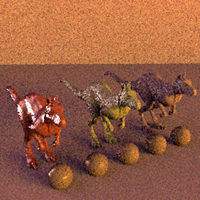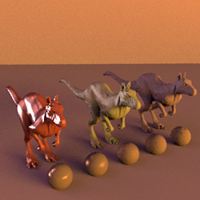Importance sampling the environment map can make an enormous difference in the quality of the rendered image. Both images below are rendered using the same number of samples. In the image below at left, samples are drawn from a cosine-weighted distribution centered about the normal of the surface being shaded (see lights/infinite.cpp). However, in the case of an infinite area light, we know the light's luminance ahead of time, and therefore we can use this information to perform better sampling. At right, samples are drawn using a distribution that is proportional to the luminance of the environment map (see lights/infinitesample.cpp).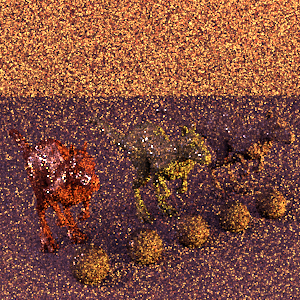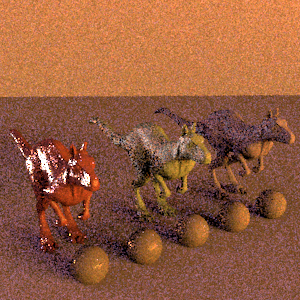Question 1. As an exercise, assume that you are given an environment map with luminance L(phi, theta) = cos(theta) when theta < PI/2 and L(phi, theta) = 0 otherwise. Write down a pdf that could be used to sample the environment with respect to luminance. Use the inversion method to find expressions for phi and theta in terms of uniform random variables x1 and x2 so that a direction on the sphere is chosen randomly according to your pdf.

Note in a contrived case such as this, integrating the pdf analytically is possible. However, in practice in pbrt, radiance from the direction (phi, theta) is given by the value of a texture map at position (u,v). In this case, to implement the inversion method, CDF must be computed numerically. This is precisely what is done by lights/infinitesample.cpp. Take the time to understand how this implementation works.